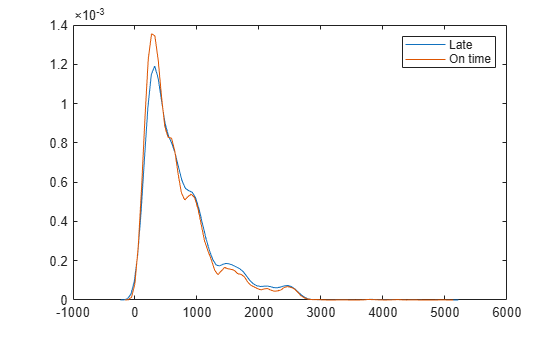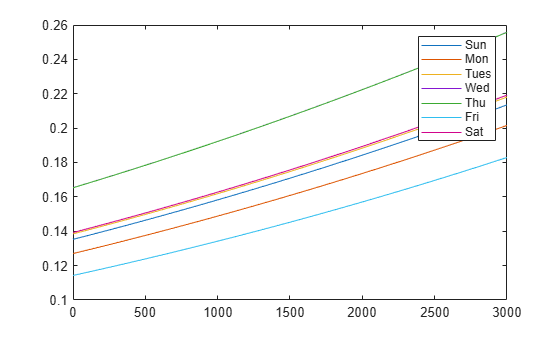Documentation

Logistic Regression with Tall Arrays

This example shows how to use logistic regression and other techniques to perform data analysis on tall arrays. Tall arrays represent data that is too large to fit into computer memory.

Define Execution Environment

When you perform calculations on tall arrays, MATLAB® uses either a parallel pool (default if you have Parallel Computing Toolbox™) or the local MATLAB session. To run the example using the local MATLAB session when you have Parallel Computing Toolbox, change the global execution environment by using the mapreducer function.

mapreducer(0)

Get Data into MATLAB

Create a datastore that references the folder location with the data. The data can be contained in a single file, a collection of files, or an entire folder. Treat 'NA' values as missing data so that datastore replaces them with NaN values. Select a subset of the variables to work with, and include the name of the airline (UniqueCarrier) as a categorical variable. Create a tall table on top of the datastore.

ds = datastore('airlinesmall.csv');
ds.TreatAsMissing = 'NA';
ds.SelectedVariableNames = {'DayOfWeek','UniqueCarrier',...
'ArrDelay','DepDelay','Distance'};
ds.SelectedFormats{2} = '%C';
tt = tall(ds);
tt.DayOfWeek = categorical(tt.DayOfWeek,1:7,...
{'Sun','Mon','Tues','Wed','Thu','Fri','Sat'},'Ordinal',true)
tt =

Mx5 tall table

DayOfWeek    UniqueCarrier    ArrDelay    DepDelay    Distance
_________    _____________    ________    ________    ________

?              ?             ?           ?           ?
?              ?             ?           ?           ?
?              ?             ?           ?           ?
:              :             :           :           :
:              :             :           :           :

Late Flights

Determine the flights that are late by 20 minutes or more by defining a logical variable that is true for a late flight. Add this variable to the tall table of data, noting that it is not yet evaluated. A preview of this variable includes the first few rows.

tt.LateFlight = tt.ArrDelay>=20
tt =

Mx6 tall table

DayOfWeek    UniqueCarrier    ArrDelay    DepDelay    Distance    LateFlight
_________    _____________    ________    ________    ________    __________

?              ?             ?           ?           ?            ?
?              ?             ?           ?           ?            ?
?              ?             ?           ?           ?            ?
:              :             :           :           :            :
:              :             :           :           :            :

Calculate the mean of LateFlight to determine the overall proportion of late flights. Use gather to trigger evaluation of the tall array and bring the result into memory.

m = mean(tt.LateFlight)
m =

tall double

?
m = gather(m)
Evaluating tall expression using the Local MATLAB Session:
- Pass 1 of 2: Completed in 0.74 sec
- Pass 2 of 2: Completed in 0.85 sec
Evaluation completed in 2 sec
m = 0.1580

Late Flights by Carrier

Examine whether certain types of flights tend to be late. First, check to see if certain carriers are more likely to have late flights.

tt.LateFlight = double(tt.LateFlight);
late_by_carrier = gather(grpstats(tt,'UniqueCarrier','mean','DataVar','LateFlight'))
Evaluating tall expression using the Local MATLAB Session:
- Pass 1 of 1: Completed in 2.5 sec
Evaluation completed in 3 sec
late_by_carrier=29×4 table
GroupLabel    UniqueCarrier    GroupCount    mean_LateFlight
__________    _____________    __________    _______________

{'9E'    }       9E                521           0.13436
{'AA'    }       AA              14930           0.16236
{'AQ'    }       AQ                154          0.051948
{'AS'    }       AS               2910           0.16014
{'B6'    }       B6                806           0.23821
{'CO'    }       CO               8138           0.16319
{'DH'    }       DH                696           0.17672
{'DL'    }       DL              16578           0.15261
{'EA'    }       EA                920           0.15217
{'EV'    }       EV               1699           0.21248
{'F9'    }       F9                335           0.18209
{'FL'    }       FL               1263           0.19952
{'HA'    }       HA                273          0.047619
{'HP'    }       HP               3660           0.13907
{'ML (1)'}       ML (1)             69          0.043478
{'MQ'    }       MQ               3962           0.18778
⋮

Carriers B6 and EV have higher proportions of late flights. Carriers AQ, ML(1), and HA have relatively few flights, but lower proportions of them are late.

Late Flights by Day of Week

Next, check to see if different days of the week tend to have later flights.

late_by_day = gather(grpstats(tt,'DayOfWeek','mean','DataVar','LateFlight'))
Evaluating tall expression using the Local MATLAB Session:
- Pass 1 of 1: Completed in 1.3 sec
Evaluation completed in 1.7 sec
late_by_day=7×4 table
GroupLabel    DayOfWeek    GroupCount    mean_LateFlight
__________    _________    __________    _______________

{'Fri' }       Fri          15839           0.12899
{'Mon' }       Mon          18077           0.14234
{'Sat' }       Sat          16958           0.15603
{'Sun' }       Sun          18019           0.15117
{'Thu' }       Thu          18227           0.18418
{'Tues'}       Tues         18163           0.15526
{'Wed' }       Wed          18240           0.18399

Wednesdays and Thursdays have the highest proportion of late flights, while Fridays have the lowest proportion.

Late Flights by Distance

Check to see if longer or shorter flights tend to be late. First, look at the density of the flight distance for flights that are late, and compare that with flights that are on time.

ksdensity(tt.Distance(tt.LateFlight==1))
Evaluating tall expression using the Local MATLAB Session:
- Pass 1 of 2: Completed in 0.9 sec
- Pass 2 of 2: Completed in 0.75 sec
Evaluation completed in 1.9 sec
hold on
ksdensity(tt.Distance(tt.LateFlight==0))
Evaluating tall expression using the Local MATLAB Session:
- Pass 1 of 2: Completed in 0.92 sec
- Pass 2 of 2: Completed in 1.4 sec
Evaluation completed in 2.6 sec
hold off
legend('Late','On time')Flight distance does not make a dramatic difference in whether a flight is early or late. However, the density appears to be slightly higher for on-time flights at distances of about 400 miles. The density is also higher for late flights at distances of about 2000 miles. Calculate some simple descriptive statistics for the late and on-time flights.

late_by_distance = gather(grpstats(tt,'LateFlight',{'mean' 'std'},'DataVar','Distance'))
Evaluating tall expression using the Local MATLAB Session:
- Pass 1 of 1: Completed in 1.5 sec
Evaluation completed in 2 sec
late_by_distance=2×5 table
GroupLabel    LateFlight    GroupCount    mean_Distance    std_Distance
__________    __________    __________    _____________    ____________

{'0'}           0          1.04e+05        693.14           544.75
{'1'}           1             19519        750.24           574.12

Late flights are about 60 miles longer on average, although this value makes up only a small portion of the standard deviation of the distance values.

Logistic Regression Model

Build a model for the probability of a late flight, using both continuous variables (such as Distance) and categorical variables (such as DayOfWeek) to predict the probabilities. This model can help to determine if the previous results observed for each predictor individually also hold true when you consider them together.

glm = fitglm(tt,'LateFlight~Distance+DayOfWeek','Distribution','binomial')
Iteration :	  0% completed
Iteration :	 100% completed
Iteration :	  0% completed
Iteration :	 100% completed
Iteration :	  0% completed
Iteration :	 100% completed
Iteration :	  0% completed
Iteration :	 100% completed
Iteration :	  0% completed
Iteration :	 100% completed
glm =
Compact generalized linear regression model:
logit(LateFlight) ~ 1 + DayOfWeek + Distance
Distribution = Binomial

Estimated Coefficients:
Estimate         SE         tStat       pValue
__________    __________    _______    __________

(Intercept)           -1.855      0.023052    -80.469             0
DayOfWeek_Mon      -0.072603      0.029798    -2.4365       0.01483
DayOfWeek_Tues      0.026909      0.029239    0.92029       0.35742
DayOfWeek_Wed         0.2359      0.028276      8.343    7.2452e-17
DayOfWeek_Thu        0.23569      0.028282     8.3338    7.8286e-17
DayOfWeek_Fri       -0.19285      0.031583     -6.106    1.0213e-09
DayOfWeek_Sat       0.033542      0.029702     1.1293       0.25879
Distance          0.00018373    1.3507e-05     13.602    3.8741e-42

123319 observations, 123311 error degrees of freedom
Dispersion: 1
Chi^2-statistic vs. constant model: 504, p-value = 8.74e-105

The model confirms that the previously observed conclusions hold true here as well:

• The Wednesday and Thursday coefficients are positive, indicating a higher probability of a late flight on those days. The Friday coefficient is negative, indicating a lower probability.

• The Distance coefficient is positive, indicating that longer flights have a higher probability of being late.

All of these coefficients have very small p-values. This is common with data sets that have many observations, since one can reliably estimate small effects with large amounts of data. In fact, the uncertainty in the model is larger than the uncertainty in the estimates for the parameters in the model.

Prediction with Model

Predict the probability of a late flight for each day of the week, and for distances ranging from 0 to 3000 miles. Create a table to hold the predictor values by indexing the first 100 rows in the original table tt.

x = gather(tt(1:100,{'Distance' 'DayOfWeek'}));
Evaluating tall expression using the Local MATLAB Session:
- Pass 1 of 1: Completed in 0.24 sec
Evaluation completed in 0.53 sec
x.Distance = linspace(0,3000)';
x.DayOfWeek(:) = 'Sun';
plot(x.Distance,predict(glm,x));

days = {'Sun' 'Mon' 'Tues' 'Wed' 'Thu' 'Fri' 'Sat'};
hold on
for j=2:length(days)
x.DayOfWeek(:) = days{j};
plot(x.Distance,predict(glm,x));
end
legend(days)According to this model, a Wednesday or Thursday flight of 500 miles has the same probability of being late, about 18%, as a Friday flight of about 3000 miles.

Since these probabilities are all much less than 50%, the model is unlikely to predict that any given flight will be late using this information. Investigate the model more by focusing on the flights for which the model predicts a probability of 20% or more of being late, and compare that to the actual results.

C = gather(crosstab(tt.LateFlight,predict(glm,tt)>.20))
Evaluating tall expression using the Local MATLAB Session:
- Pass 1 of 1: Completed in 1.2 sec
Evaluation completed in 1.3 sec
C = 2×2

99613        4391
18394        1125

Among the flights predicted to have a 20% or higher probability of being late, about 20% were late 1125/(1125 + 4391). Among the remainder, less than 16% were late 18394/(18394 + 99613).